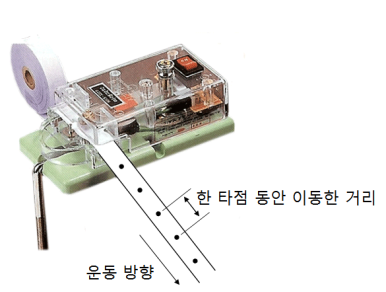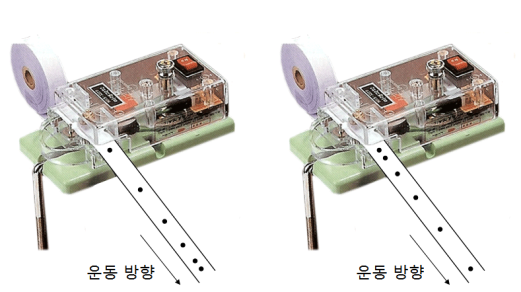# Ticker Timer

Ticker Timer

It is a device to record the movement of an object by taking a spot on a paper tape at regular time intervals. A typical ticker timer is plugged into a household outlet. So the ticker cycle of the ticker timer is closely related to the frequency of the supplied household power.If 60Hz power is supplied, the ticker timer will also take 60 points per second. Therefore, the period per spot can be calculated as follows.

period(s) = 1 / frequency(Hz) = 1/ 60 ≒ 0.167

Velocity is calculated by dividing the distance traveled by time.

Velocity(m/s) = distance(m) / time(s) = distance(m) / 0.167(s)

Analyzing the ticker timer

The ticker timer records the movement while pulling the paper tape. Therefore, the recent movement is recorded on the tape near the ticker timer. Please refer to the figure below for how to analyze the ticker timer.Slow constant motion(left) and fast constant motion(right)Increasingly fast (left) and increasingly slow (right)